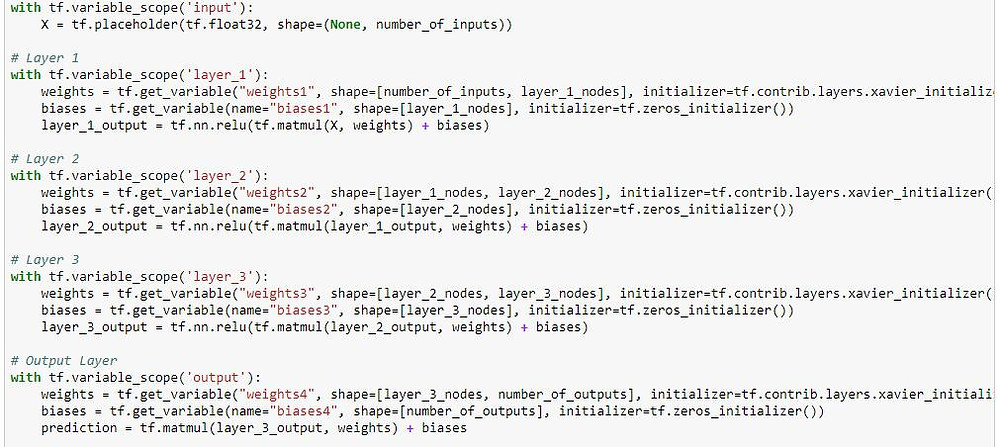# Code a Google TensorFlow Artificial Neural Network in 10 minutes with this blogThis blog will teach you to get moving and create your first neural network in 10 minutes. We will use the Google Open source deep learning platform called as TensorFlow for this.

To install and have an overview of TensorFlow have a look at these blogs from MieRobot

Google TensorFlow installation in Windows using Anaconda

What is Anaconda and why you need it for Machine/Deep learning

Installation of Scikit Learn on your laptop

Our overall goal is to create a 3 layer neural network and get this to train on a dataset. We then use the trained model to check our predictions. We will use 'TF' as TensorFlow in the rest of the blog.

First, let us test that all components of TensorFlow are working by doing a simple addition.

We also turn off the logs at 2 but this is an optional step.

Each TF would need a Session to compute and we call the Addition in a session as -

result = session.run(addition, feed_dict={X: [11, 12, 410], Y: [24, 12, 310]})The two input tensors are hard-coded just for testing purpose. We run this and we see the Addition result. So all looks good for setting up the Neural network.

Our datasheet is a Sales data sheet whose values if you see closely are in Lower range of 0 and 1 only. This may cause problems with our ANN and hence we need to Scale input values for TF using a standard function from SciKit learn.

For any ANN we can have 3 ways to input data in a model. They are - a) Using in memory (make sure your computer RAM size is >> Input file size), b) Use Data Pipes for very data sets as images and videos c) Use code to split step by step. We will use the simple method of using in memory using Pandas data frame.

The input files are dummy here and assume the column headers A,B,C,D stands for some business needs and is confidential. In many real life cases while working client will probably not say what columns stand for. The predicted value would be the column total_earnings.

We use the MinMaxScaler from the famous Scikit learn package. MinMaxScaler Transforms features by scaling each feature to a given range. It may multiply or add some random numbers to change values. It is no brainer that we take the predicted column name (total_earnings) from the X train as drooping column. If this is new to you please check on train-test-split. Here we have done the spilt using separate input files for training and testing.This estimator scales and translates each feature individually such that it is in the given range on the training set, i.e. between zero and one.

The documentation can be seen at the link here at:

http://scikit-learn.org/stable/modules/generated/sklearn.preprocessing.MinMaxScaler.html

We then Pull out columns for X (data to train with) and Y (value to predict). This is pretty much standard after that but be sure that the training and test data are scaled with the same scaler.

Here is how it looked for me as an output:Ok Go ahead and run now. You would see the Scaler working to get you scaled values.

Now - we would need to train the model.

We frame the Neural network as with below parameters for 100 epochs with 9 Inputs and 1 output which is the Predicted Price.

# Define model parameters learning_rate = 0.001 training_epochs = 100

# Define how many inputs and outputs are in our neural network number_of_inputs = 9 number_of_outputs = 1

# Define how many neurons we want in each layer of our neural network layer_1_nodes = 50 layer_2_nodes = 100 layer_3_nodes = 50

Layer 2 is the hidden layer and it has more layers at 100. Feel free to change the layer nodes and learning rates and compare the results. One Epoch is one full run of the training set. We use the train data set to train the 3 layer model with a TF session as -

session.run(optimizer, feed_dict={X: X_scaled_training, Y: Y_scaled_training})

Go ahead and run the code. You would see the model getting trained. Take a pause now and pat yourself. You are midway through. Well done!Your result should show the training getting completed in 100 epochs with interval of 5.In the final part we would need to test the trained model using cost function.

Define the cost function of the neural network that will measure prediction accuracy during training. Then we Define the optimiser function that will be run to optimise the neural network. The code block is as below:

with tf.variable_scope('cost'): Y = tf.placeholder(tf.float32, shape=(None, 1)) cost = tf.reduce_mean(tf.squared_difference(prediction, Y))

# Section Three: Define the optimizer function that will be run to optimize the neural network

Finally, call a TF session and check for every 5 training steps, log our progress. The Model will improve and cost function will reduce showing improvement in the ANN learning outcome. The final outcome I got from the prediction value is below and you would have a similar value depending on the configurations you did.Final Training cost: 7.252251089084893e-05 Final Testing cost: 0.00011301010817987844 The actual earnings of Game #1 were \$247537.0 Our neural network predicted earnings of \$252468.328125

Note please do a reset graph if you are using Jupyter Notebook. If you are over a Python IDE like PyCharm this may not be needed. tf.reset_default_graph()

The full code can be downloaded as Python notebook at our Github at: https://github.com/MieRobot/Blogs/blob/master/tensorflow_SimpleANN_Blog.ipynb

The test files can be seen at GitHub as below:Thank you for reading and keep learning.

https://www.tensorflow.org/api_docs/python/tf/reset_default_graph

About Author: Anirban runs an EduTech startup brand called MieRobot.com which provides on-campus employability solutions in areas of Machine learning, Graph Database, Robotics and Product Management. You can say him a hello at hello@mierobot.com